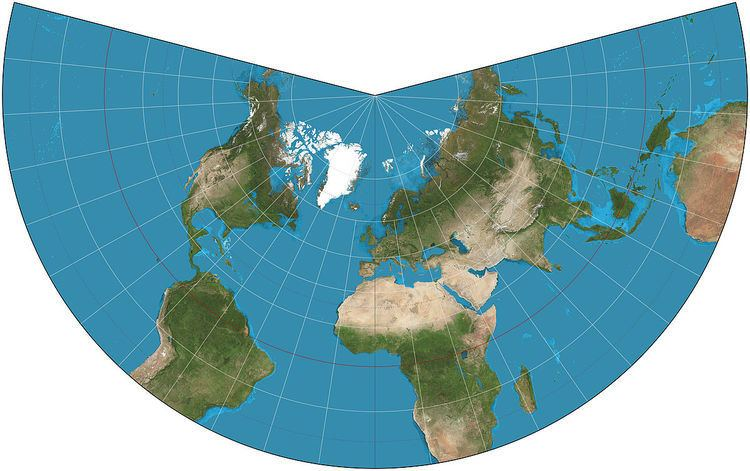# Lambert conformal conic projection

Updated onA Lambert conformal conic projection (LCC) is a conic map projection used for aeronautical charts, portions of the State Plane Coordinate System, and many national and regional mapping systems. It is one of seven projections introduced by Johann Heinrich Lambert in his 1772 publication Anmerkungen und Zusätze zur Entwerfung der Land- und Himmelscharten (German: Notes and additions for creating ground and aerial charts).

## Contents

Conceptually, the projection seats a cone over the sphere of the Earth and projects the surface conformally onto the cone. The cone is unrolled, and the parallel that was touching the sphere is assigned unit scale. That parallel is called the reference parallel or standard parallel.

By scaling the resulting map, two parallels can be assigned unit scale, with scale decreasing between the two parallels and increasing outside them. This gives the map two standard parallels. In this way, deviation from unit scale can be minimized within a region of interest that lies largely between the two standard parallels. Unlike other conic projections, no true secant form of the projection exists because using a secant cone does not yield the same scale along both standard parallels.

## Use

Pilots use aeronautical charts based on LCC because a straight line drawn on a Lambert conformal conic projection approximates a great-circle route between endpoints for typical flight distances. The US systems of VFR (visual flight rules) sectional charts and terminal area charts are drafted on the LCC with standard parallels at 33°N and 45°N.

The European Environment Agency and the INSPIRE specification for coordinate systems recommends using this projection (also named ETRS89-LCC) for conformal pan-European mapping at scales smaller or equal to 1:500,000. In Metropolitan France, the official projection is Lambert-93, a Lambert conic projection using RGF93 geodetic system and defined by references parallels that are 44°N and 49°N.

The National Spatial Framework for India uses Datum WGS84 with a LCC projection and is a recommended NNRMS standard. Each state has its own set of reference parameters given in the standard.

The U.S. National Geodetic Survey's "State Plane Coordinate System of 1983" uses the Lambert conformal conic projection to define the grid-coordinate systems used in several states, primarily those that are elongated west to east such as Tennessee. The Lambert projection is relatively easy to use: conversions from geodetic (latitude/longitude) to State Plane Grid coordinates involve trigonometric equations that are fairly straightforward and which can be solved on most scientific calculators, especially programmable models. The projection as used in CCS83 yields maps in which scale errors are limited to 1 part in 10,000.

## History

The Lambert conformal conic is one of several map projection systems developed by Johann Heinrich Lambert, an 18th-century Swiss mathematician, physicist, philosopher, and astronomer.

## Transformation

Coordinates from a spherical datum can be transformed into Lambert conformal conic projection coordinates with the following formulas, where λ is the longitude, λ0 the reference longitude, φ the latitude, φ0 the reference latitude, and φ1 and φ2 the standard parallels:

x = ρ sin [ n ( λ λ 0 ) ] y = ρ 0 ρ cos [ n ( λ λ 0 ) ]

where

n = ln ( cos φ 1 sec φ 2 ) ln [ tan ( 1 4 π + 1 2 φ 2 ) cot ( 1 4 π + 1 2 φ 1 ) ] ρ = F cot n ( 1 4 π + 1 2 φ ) ρ 0 = F cot n ( 1 4 π + 1 2 φ 0 ) F = cos φ 1 tan n ( 1 4 π + 1 2 φ 1 ) n

Formulae for ellipsoidal datums are more involved.

If one standard parallel is used (i.e. φ1 = φ2), the formula for n above is indeterminate, but then n = sin(φ1).

Topics